To find center, the unique point in 2 or higher-dimensional space

Author: Yumnam Kirani Singh
Member Calcutta Mathematical Society
Email: kiranisingh@hotmail.com

Some formulae for finding the centre of a circle or sphere or in general hypersphere, which will pass through some given number of points are given here. We can draw infinite number of circles, which can pass through one or two given points. But if the number of points given is three, then we can draw only one circle, which will pass through the three given points. Also, we can draw many spheres, which will pass through any three or less number of given points. But we can draw only one sphere, which will pass through any four given points in 3-dimension. Similarly, we can find a unique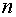-dimensional hypersphere, which will pass through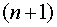given points.
In this paper, we also show the relation between a circle and a triangle. Some interesting properties of triangles are also briefly mentioned here, which may be useful from computational geometry application point of view. All the formulae given here can be obtained easily with some knowledge of analytical co-ordinate geometry and hence the derivations are not shown.
We will define points inCartesian co-ordinates. By a two dimensional point we will mean a point which requires two co-ordinates to locate it exactly. Similarly by a three-dimensional point, we mean a point whose exact location requires three co-ordinates and so on.

Formula 1: The co-ordinates of a pointon the perpendicular bisector of two given pointsat a distance d from the mid-point of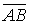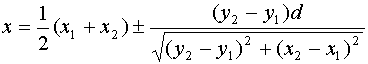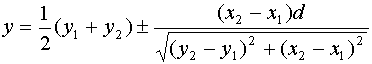In the above formulas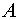indicates there are two possible values for each ofand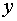i.e., on the both sides of the line joiningandFrom the above formulae, we can see that when we draw a circle withas the centre, the circle will always pass through the two pointsand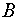. As we can have infinite values of d, we will obtain infinite number of centres, i.e., infinite numbers of circles which will pass through the two points.

Formula 2: Let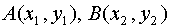and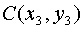be any three points, then there exists a unique point, equidistant from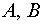andwhose co-ordinates are given by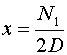,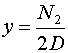Where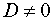Andare given by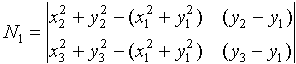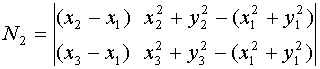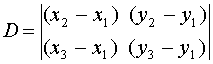From formula 2, we know that there is a unique point in 2-dimensional plane, which is at equal distances from any three given points in the plane. In other words we can say for every triangle there exists a unique circle, which can exactly circumscribe the triangle. Therefore, there cannot be two or more circles, which can exactly circumscribe a given triangle. So triangles and circles are closely related. In other words we can rightly say, ‘ A triangle is a plane figure formed by joining any three points on the circumference of a circle’, or, we can say the vertices of a triangle are any three points on a circumference of a circle.
We know a triangle is closely related to a circle. If the vertices of a triangle are given, then we can easily determine the centre of the circle, which will circumscribe the triangle using formula 2. Otherwise we have to use some geometrical means to find the centre. Now we describe a simple geometrical means to find the center of a circle, which will circumscribe a given triangle, as follows.

Property-1: The bisectors of the three sides of a triangle always intersect at the center of the circle, which circumscribe it.

Property 2: The point of intersection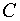, of the bisectors of the two smaller sides of a triangle gives the center of the circle, which will circumscribe it.is also known as Voronoi vertex of the triangle.
This property can be used for computing Delauney triangulation and Voronoi diagram as given below.

Drawing Delauney Triangulation: Draw triangles in such a way that there exists no other point whose distance from, the point of intersection of the bisectors of the two smaller sides of a triangle, is less than the distance offrom any of its vertices.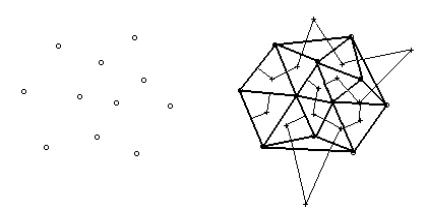Fig-2. Delauney Triangulation.

In figure-2, the thick lines give the Delauney tringulation. The thin lines are the bisectors of the two smaller sides of every triangle in the triangulation structure. The + indicates the point of intersections, i.e., Voronoi Vertex of each associated triangle.

Drawing Voronoi diagram:Join the adjacent Voronoi vertices in Fig-2. And draw rays assumed to be emanated from the mid-points of the outermost sides of the Delauney triangulation.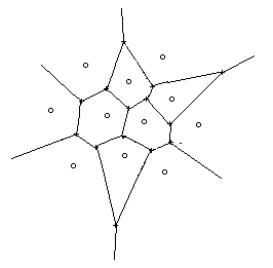Fig-3. Voronoi Diagram of the points in Figure-1.

The proof of property 1 and property 2 can be done easily using formula 1.
So by simply using property 2, we can easily find the centre of a circle that circumscribes a given triangle. As we know a polygon can be decomposed into triangles, we can say whether there can be a circle that passes through the vertices of apolygon or not.
Also we can say, whether a given triangle is an acute angled, right angled or an obtuse angled triangle, using property 2.

If the point of intersection of the bisectors of the two smaller sides of a triangle lies
(i) inside the triangle, then the triangle is an acute angled triangle.
(ii)on the middle of the third side, then the triangle is a right angled triangle.
(iii)outside the triangle, then the triangle is an obtuse angled triangle.

This property can be used for computing the minimum enclosing circle of a given set of points.

Formula 3: Letand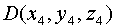be any four points ,then there exists a unique pointequidistant fromand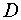whose co-ordinates are given by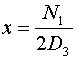,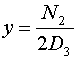and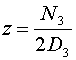provided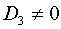where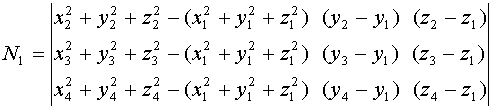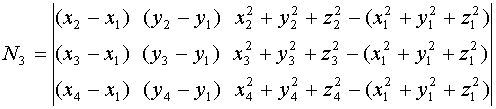andThis formula gives us the centre of the sphere, which will pass through any four given points in 3-dimension. This can be used for finding Delauney triangulation and Voronoi diagram of a set of points in 3-dimension. For any three or less number of points in 3-dimension, we can find infinite number of spheres which will pass through them, as we can draw infinite number of circles which will pass through any one or two given points.
Proceeding in this way we can find the centre of a unique n-dimensional hypersphere, which will pass through any givenpoints in n-dimensions.

Formula 4: Let,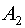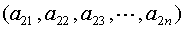,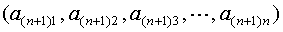be any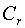given points in n-dimensional space, then there exists a unique pointwhich is equidistant from all the given points whose co-ordinates are given by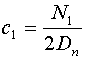,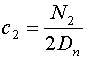,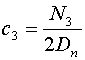,...,provided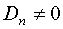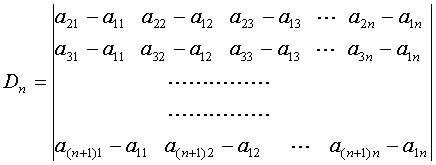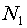=first column of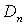being replaced by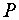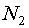=second column ofbeing replaced bycontinuing this way,column ofbeing replaced byand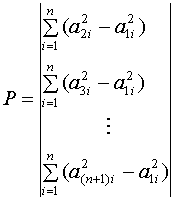This can be used for finding the centre of the n- dimensional hypersphere for any given (n+1) points. This centre is unique for the given (n+1) points. If the number of points in n-dimensions is less than or equal to n, then we can find infinite number of n-dimensional hypersphere, which will pass through the given points. This can also be used for finding the Delauney tringulation and Voronoi diagram in n-dimension.

References:

Franco P Preparata, M. I. Shamos, (1985), Computational Geometry :An Introduction

G. Toussaint ,(1985), Edt., Computational Geometry,Elsevier Science Publishers, B.V.

``` Published in News Bull. Cal. Math. Soc. 24(5&6) 21-24(2001). ```